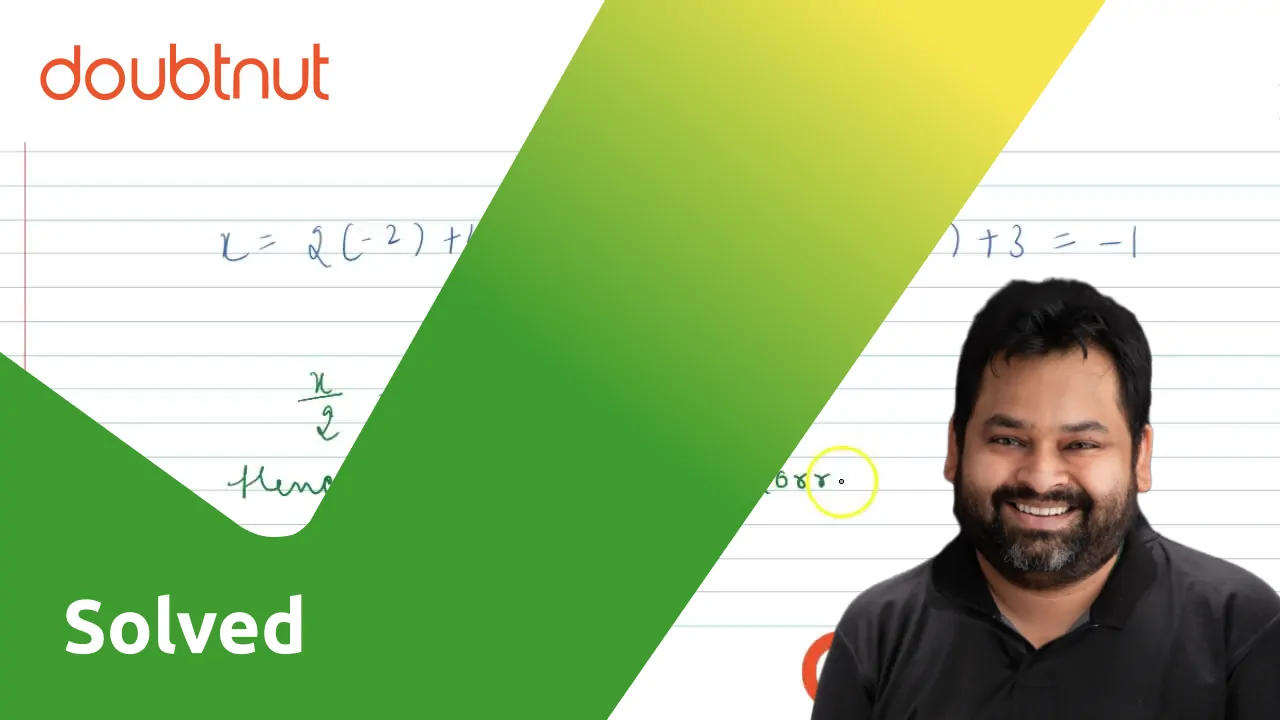Doubtnut App - Get 50 Lakh+ video solutions better than GoogleInstall Now

# Which of the following line is passing through point of intersection of line x−42=y−32=z−51 and the plane x+y+z=2 (a) x2=y+13=z−34 (b) x−23=y−13=z−32 (c) x2=y+13=z−24 (d) x−22=y+13=z+34

Video Solution